﻿ 520.490 Analog and Digital VLSI Systems: ADM Speech Encoder

# Adaptive Delta Modulation for Speech Encoding

 Stephen Ajemian sajemia1@jhu.edu Richard Hamilton rhamilt1@jhu.edu John Truelove jtruelove@jhu.edu Mike Poston mcp15@jhu.edu

acelik@jhu.edu## Objectives

Delta Modulation (DM) is an analog-to-digital conversion used for transmitting analog signals by simply transmitting the difference of an input signal to a reference signal. This difference, as determined by a comparator, is represented by a "0" or a "1", which in this implementation is 0 V and 3 V respectively. A 0 V comparator output indicates that the input signal is less than the approximated signal, whereas a 3 V output indicates that the input signal is greater than the approximated signal. The reference signal consists of the sum of all previous differences between these two voltage signals. However, a significant issue exists in using delta modulation for the encoding of analog signals. The DM is unable to track rapid changes in the amplitude of the input signal giving a distorted demodulated signal. Should an input signal vary rapidly over time, slope overload occurs. As the modulated signal is transmitted and recovered, it will demonstrate large amplitude variations from the input signal. A solution to this slope overload issue is an adaptive delta modulator (ADM). In this implementation of an ADM, slope overload is eliminated by using digital logic to adjust the step size of the integrator used to construct the reference voltage signal. By using previous values of the differences between the two voltage signals, the digital logic will increase or decrease the step size to provide more accurate tracking of the input voltage signal.## Specifications

The following table outlines the pin connections for our ADM circuit. Depending on the input signal, certain values must be optimized to obtain an accurate output from our circuit. The rail voltages, Vrail+ and Vrail-, are the basic building blocks of the integrator used to track the input signal. These values are the values that are ampified and fed back to the comparator. Values for these voltages are signal dependent. Other signal dependent values are the clock input to the cicuit, and the bias voltage for the low pass filter. For the clock input, one must consider the frequency of the input signal. For our simulations, we sampled the input at about 40 times the Nyquist rate. (Input frequency x 2) Therefore, in order to obtain useful output, one must choose a clock speed appropriate to the input signal so as to achieve accurate resolution in the output signal.

 1 Demodulator Integrator Output 40 Integrator Positive Rail Voltage (V+) 2 39 Integrator Negative Rail Voltage (V-) 3 Demodulator Final Output (Vout) 38 4 Demodulator Low-Pass Filter Bias V 37 Demodulator CNT DIR 5 Gnd 36 6 35 7 34 8 33 9 Demodulator Sample/Hold Output 32 Demodulator Input (Vin) 10 Analog Vdd 31 Clock Input 11 Modulator Integrator Output 30 Clock 1 (output) 12 Modulator Vin 29 Clock 2 (output) 13 28 Modulator CNT DIR 14 27 15 Vdd (Digital) 26 16 25 17 Vpup – Inv Amp Bias 24 18 Vpdown – Inv Amp Bias 23 19 Vn – Inv Amp Bias 22 20 Modulator Comparator Output (Vout) 21

Circuit Overview
Shown below is a high-level schematic of our circuit. The circuit consists of four basic components: the two-phase clock generator, modulator, demodulator, and low-pass filter. The ADM circuit is implemented this way to ensure a modular design that we could debug piece-wise.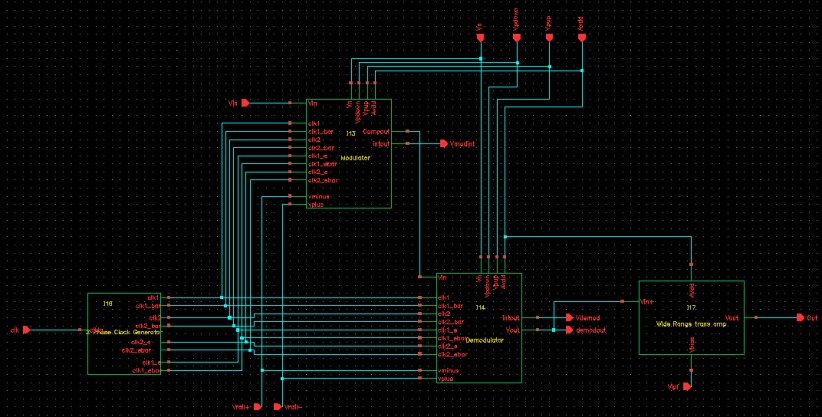Two-Phase Clock Generator
The two phase clock generator takes a single clock pulse as input, and generates two non-overlapping clock pulses, clk1 and clk2, to be used throughout the cicuit. We also generate a clk1_e and clk2_e, which are earlier versions of clk1 and clk2. These clock pulses have a falling edge before clk1 and clk2, respectively. These "early" clocks are neccessary for our S/H implementations in the various parts of the ADM circuit. Our comparators and integrators are sample and hold implementations, and the clock pulses must be generated on chip to ensure non-overlapping clocks, and dependable "early" clock pulses. The layout for this implementation is also shown; its dimensions are about 150x500 lambda.Modulator
The purpose of the modulator (schematic and layout shown below) is to generate an output representing the difference between the input signal (Vin) and the integrator approximation (Vapp). This output can take one of two forms, either a logical "0" or "1" represented by gnd and Vdd. If Vin > Vapp, the comparator will output a 1, and if Vin < Vapp, a 0 will be sent. This comparator output is only valid during clk2, after the comparator has sampled both inputs. In addition to being transmitted, the comparator's output is input into the integrator where it is used to create the signal approximation. If a 1 is input, Vapp increases and if a 0 is input Vapp decreases. Vapp is finally fed back into the comparator where it is used to determine the next digital output.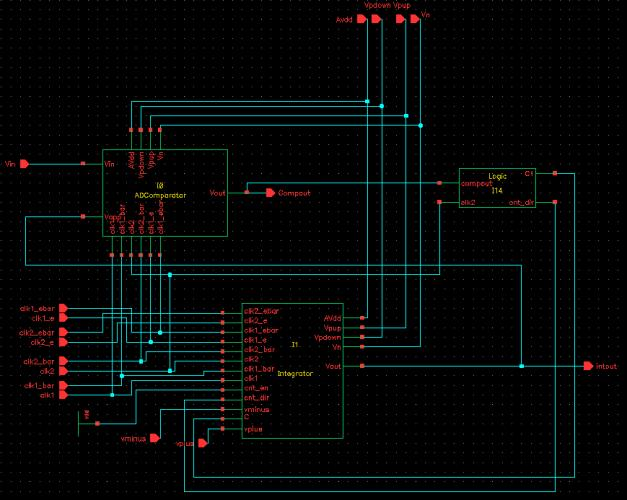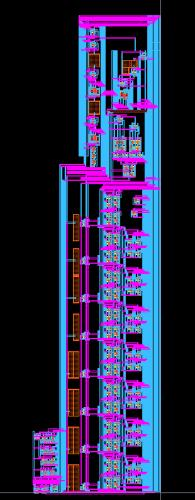Comparator
We implemented a cascoded S/H comparator using our non-overlapping two-phase clock pulses as the sample and hold signals. First, the comparator is reset to a subthreshold offset voltage using the clk1_e pulse. Next, the clk1 pulse is used to sample Vin. Since the inverting amplifiers are shorted, the voltage Vin-Voffset charges at both capacitors. Next, the clk1 pulse is released and the hold is asserted through clk2. Vapp-Voffset charges the first capacitor, then is passed through the first inverting op-amp to charge the voltage Vin-Vapp at the second capacitor. This voltage is passed through the second inverting op-amp to induce Vapp-Vin as the output voltage of the second op-amp. This voltage will be inverted thrice, which means that if Vapp-Vin is positive a digital 0 will be output and if Vapp-Vin is negative a digital 1 will be output.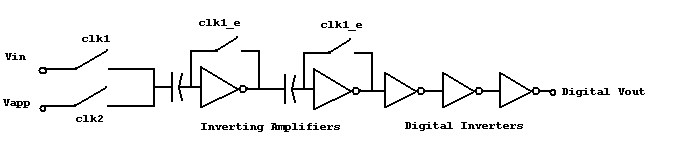Integrator
For our integrator, we used a similar S/H implementation. For our voltage precharge we are provided with two opposite rail voltages. Depending on our comparator value, we will either precharge the positive or negative rail voltage. We implement a pass-gate multiplexor with the comparator output selecting the appropriate rail voltage. The opposite voltage is then sampled, and inverted through the inverting amp to provide either positive or negative feedback. First, the clk1_e signal clears the integrator voltage and shorts the inverting amp so that when clk1 is asserted, the appropriate rail voltage is charged on C1. Next, the clk2_e signal is asserted, which precharges C2 so that when clk2 is asserted, the opposite rail voltage passes through the inverting amp and a gain of C1/C2 is added or subtraced from the voltage at C2, depending on the value of the comparator. Clearly, if the value of C1 remains constant, there will be a constant gain and therefore a constant step size in our waveform. Additionally the comparator output is only available during clk2, although we need it during both clock signals to ensure the correct rail voltage is selected. We resolve both of these issues using a sequential logic unit.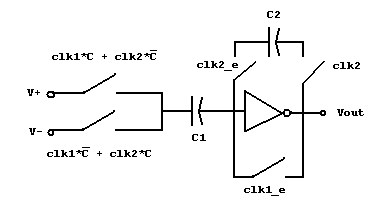After experimenting with simulations of several different adaptive logic schemes we realized that the simplest logic scheme works the best. If the comparator value is different from the one immeadiately before it, it means our input signal and our approximation have crossed each other and we need to change the direction of the approximation. In order to minimize over-correction, we want to decrease the step size. Conversely, if our comparator value is the same as the one immeadiately before it, it means that our signals have not crossed and that we want to increase the approximation's step size so as to minimize slope overload. This can be implemented very easily; with a latch to save the previous value of the comparator output and an XNOR gate to check if the current and previous values are equal.We implemented different step sizes by putting an array of 8 parallel, switched capacitors in place of C1 in the integrator. To control the switches to each capacitor we implemented an 8-bit temperature counter, with each bit in the counter driving a switch to a capacitor. Since the progression of the temperature counter is 00000001, 00000011, 00000111, ..., we simply pass a count direction bit; if cnt_dir = 1, each bit takes the value of the next least significant bit and if cnt_dir = 0, each bit takes the value of the next greater significant bit. This way, we ensure that the step size will either grow by 1 degree or shrink by 1 degree. Additionally we ensure that the least significant bit will always equal 1 so that there will always be at least a unity gain for the step size.Timing Nuances
 Finally, we need to connect all of our circuit elements such that we have all outputs available when they are needed, while being careful to optimize delay time between the output of the comparator and the output of the integrator. First, we need to make the output of the comparator available during both clock cycles, so we immediately input the comparator output into a D flip flop sampling on clk2. This way, we have this information available during the entire subsequent clock cycle. This flip flop gives us a memory unit to input into our XNOR, so we simply connect the two inputs of the XNOR to the input and output of the D flip flop. Since the input of the D flip flop is only valid during the clk2 state, this means that the output of the XNOR gate will only be valid during the clk2 state. This will work with our counter, but we have to sample our counter memory elements with the clk2 signal instead or the clk1 signal, which is used as our sample on all other elements of the chip. Since the counter takes a clock cycle to update, this means that the counter output that we have just evaluated has since been charged into the D flip flop. This is optimal because we need to input a latched signal into the integrator anyway. We essentially pipeline our counter update and our comparator output latch so that on the following clk1 the counter is ready to be precharged and on the following clk2 we have a usable integrator output to be fed back into the comparator.Demodulator
Our demodulator basically follows the same circuit implementation of our integrator. The difference is that the demodulator's integrator takes a digital input off chip, whereas the modulator uses feedback from the comparator to input to the integrator. The demodulator integrator will generate the same signal approximation as the modulator, but it must have additional elements to fully restore the input signal. We use a S/H element so that the integrator output is usable during both clock cycles, and not just clk2. The voltage in the S/H element is then passed to a first-order low pass filter, with a bias voltage controlled off chip. This eliminates the high-frequency voltages caused by the integrator approximation, and thus smoothes demodulated output.

Sample and Hold

The S/H circuit samples the integrator output voltage during the clk2 cycle, and holds this voltage during the clk1 cycle. Because the integrator output is only valid during clk2, we must extend this to both clk cycles to output an accurate representation of the signal. The low pass filter is now able to filter the integrator output over both clock cycles, thus providing a smoother output waveform to closely match the original input signal.## Results

For our first simulation, shown below, we applied a sine wave input of frequency 2 KHz. The input voltage range ran from 0.5 V to 2.5 V, the operating range of our ADM. So, the message signal took the form: m(t) = sin(4000*pi*t) + 1.5. With this 2 KHz input frequency, we chose a sampling frequency of about 167 KHz. (Approximately 42 times the Nyquist sampling rate). We sampled the input signal over several periods, and also included a plot of the demodulated output prior to the low-pass filter.

From the filtered output waveform, one can see that the output signal is an accurate approximation of the input signal. The under-approximation that occurs at startup is due to the zero initial condition of the integrator. The integrator must first build up the approximated signal to the input voltage. However, once the approximated signal reaches a value that can accurately track the input signal, it will track it quite closely. The difference in phase of the output signal as compared to the input signal can be attributed to the inherent delay of the ADM circuit. Our implemenation requires a two phase clock for the precharging that must occur within the comparator and integrator, which contributes substantially to the overall delay of the circuit. Essentially, the comparator samples the input during clock cycle 1, and its output is valid during clock cycle 2. This value is then latched for the next clock cycle 1, which is then used by the integrator during clock cycle 2. So, the generation of a valid output to be sent to the demodulator takes two clock cycles. (Based on the input to the two phase clock generator) A plot of the basic sine wave superimposed on the output signal is also included below. From the plot, one can see the minor phase shift between the input and output signals.

For our second simulation, we applied an input signal consisting of the sum of three sine waves of varying frequency. The input signal took the form of m(t) = sin(4000*pi*t) + sin(3000*pi*t) + sin(2000*pi*t) + 1.5 V. The output waveform is an accurate approximation of the input, as with the case of the basic sine wave.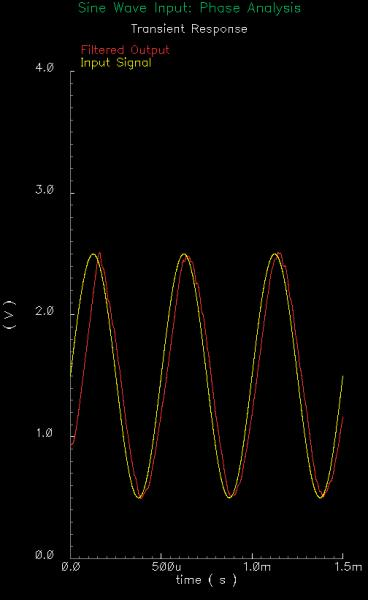In practice, the demodulator and modulator would not reside on the same chip. The comparator output will be sent through some channel to be received by the demodulator, as Delta Modulation is communication system. In this circuit, the demodulator is included to provide a glimpse of what the reconstructed signal will look like. The demodulator will take the output of the comparator from the modulator, which is either a binary "0" or "1" (0 V or 3 V), and assembles an approximation based solely on this binary input. Based on the comparator input, the demodulator uses digital logic to determine if the integrator must adjust the step size of the integration. (The integrator in the demodulator is different than the modulator integrator) This is accomplished by connecting more capacitors in parallel, thus increasing the step size. The integrator provides eight different step sizes (8 capacitors in parallel), and these capacitors are switched on or off, depending on the digital logic.

A more comprehensive simulation of the adaptibility of our ADM is shown below. For this plot, the waveform is the original sine wave input, zoomed in to the first peak of the waveform. The startup of the approximation is a very good example of the adaptibility. One can see that the integrator is trying to "catch up" with the input waveform, and the step size quickly increases to the maximum step. As soon as the integrator outputs an approximation greater than the input signal, the approximation will climb back down. However, for this descent, the step size will be decremented because the input signal must be between these two approximations. The digital logic will also change the step size if two consecutive comparator outputs are the same. At the peak of the input waveform, notice that the approximation is oscillating above and below the input signal. This oscillation will decrement the step size everytime the comparator output changes, and the approximation will track the input signal accurately for a slow changing input.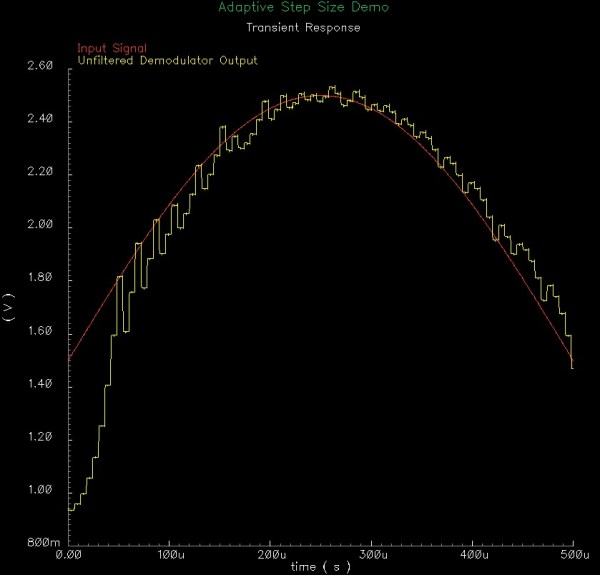## References

Lathi, B. P., Modern Digital and Analog Communications Systems, Oxford University Press, 1998.